Pramreiter, M., Stadlmann, A., Linkeseder, F., Keckes, J., and Müller, U. (2020). "Non-destructive testing of thin birch (Betula pendula Roth.) veneers," BioRes. 15(1), 1265-1281.

#### Abstract

In technical applications of wood-based composites, the predictability of elasticity and strength is important. The aim of this study was to predict the static modulus of elasticity and tensile strength of thin (0.55 mm ± 0.05 mm) birch veneers. Based on the dynamic modulus of elasticity estimated via means of wave transmission time the observed dynamic modulus of elasticity was on average 14% lower than the corresponding static modulus of elasticity. This difference could be explained by a decreased measuring area during the tensile testing or by defects within the samples. The dynamic modulus of elasticity correlated well with the static modulus of elasticity (r = 0.821). Therefore, using wave transmission time to non-destructively predict the elasticity of veneers proves to be a promising tool. The dynamic modulus of elasticity showed a significant and positive correlation with the tensile strength (r = 0.665), but this correlation was weaker than with the static modulus of elasticity. Therefore, the wave transmission time or the static modulus of elasticity must be combined with additional strength-influencing properties, such as fiber angle or density, to allow for a highly accurate prediction of tensile strength.

Non-destructive Testing of Thin Birch (Betula pendula Roth.) Veneers

Maximilian Pramreiter,a,* Alexander Stadlmann,a Florian Linkeseder,a Jozef Keckes,b and Ulrich Müller a

In technical applications of wood-based composites, the predictability of elasticity and strength is important. The aim of this study was to predict the static modulus of elasticity and tensile strength of thin (0.55 mm ± 0.05 mm) birch veneers. Based on the dynamic modulus of elasticity estimated via means of wave transmission time the observed dynamic modulus of elasticity was on average 14% lower than the corresponding static modulus of elasticity. This difference could be explained by a decreased measuring area during the tensile testing or by defects within the samples. The dynamic modulus of elasticity correlated well with the static modulus of elasticity (r = 0.821). Therefore, using wave transmission time to non-destructively predict the elasticity of veneers proves to be a promising tool. The dynamic modulus of elasticity showed a significant and positive correlation with the tensile strength (r = 0.665), but this correlation was weaker than with the static modulus of elasticity. Therefore, the wave transmission time or the static modulus of elasticity must be combined with additional strength-influencing properties, such as fiber angle or density, to allow for a highly accurate prediction of tensile strength.

Keywords: Dynamic modulus of elasticity; Elastic properties; Static modulus of elasticity; Strength; Stress wave propagation

Contact information: a: Institute of Wood Technology and Renewable Materials, Department of Material Science and Process Engineering, University of Natural Resources and Life Sciences, Vienna (BOKU), Konrad Lorenz Strasse 24, Tulln an der Donau A-3430, Austria; b: Erich Schmid Institute of Materials Science of the Austrian Academy of Sciences, Jahnstrasse 12, 8700 Leoben, Austria;

* Corresponding author: maximilian.pramreiter@boku.ac.at

INTRODUCTION

Every technical load-bearing application of wood, either sawn timber or engineered wood products (EWPs), requires knowledge about their mechanical behavior. In the case of EWPs, the mechanical properties are heavily influenced by the wood elements, their orientation in the composite, and the adhesive bonding of the elements (Hwang and Komatsu 2002). New fields of wood applications demand the knowledge of the characteristic values and the predictability of the mechanical material behavior of EWPs and wood hybrid materials (Müller et al. 2019a).

Numerical methods are based on a valid material model, characterizing the elastic, plastic, and fracture behavior of the material and on material cards, describing the characteristic values of the material. The characteristic values for describing the elastic behavior are as follows: the modulus of elasticity, the shear modulus, and the Poisson’s ratio. There are several methods described in different standards for measuring the modulus of elasticity. Driven by the demand for the shear moduli (Tschopp 2012) and Poisson’s ratio (Kumpenza et al. 2018) of different wood species for finite element modelling (FEM), current research work is dealing with the reproducible measurement of these characteristic values. Non-destructive testing provides a valuable contribution for collecting data for these characteristic values.

Numerical methods such as FEM are state-of-the-art for structural engineering and are increasingly used in timber engineering. Current research work tried to introduce wood into the mobility sector (Müller et al. 2019b). Müller et al. laid their focus on FEM of automotive parts using EWPs and wood hybrid components. Additional investigations concern other ways of transport including touring coaches, snow mobiles, and concept vehicles. The prediction of the performance of vehicle elements in static, dynamic, and crash situations demanded accurate and reliable data on the elastic, plastic, and fracture behavior of the materials used (Jost et al. 2018).

For mechanical and automotive engineering and other advanced applications of wood products, the predictability and grading of the strength and stiffness properties of the material was demanded for an appropriate safety concept. However, the prediction of the mechanical properties of every single layer of a laminated element gave the opportunity for the production of efficient tailor-made components, which can be designed with state-of-the-art computer aided methods. Knowledge of the mechanical properties of each individual layer opens up new potential for the optimum internal layer structure of plywood and laminated wood elements in terms of reliability (i.e. improved safety concept), material use (e.g. low-quality veneers or layers in the middle) and mechanical properties (i.e. flawless high-strength veneers or layers in the face layers).

Wood is a natural material, and different species show a variability in mechanical properties. Different safety concepts exist to consider the material variability. For structural engineering the general safety concept considers internal stresses from external loads and ensures that they can be absorbed without failure of the component (Wittel et al. 2017). Different material grades are considered in the safety concept of structural engineering of wooden structures, especially in the standard ÖNORM EN 1995-1-1 (2019). Non-destructive determination of the dynamic modulus of elasticity (dMOE) is one of the pillars for wood sorting and grading (Ross 2015). A strong correlation between the static and dynamic modulus of elasticity of solid wood and sawn timber was shown by different researchers (Ilic 2003; Sonderegger et al. 2008). In the area of non-destructive testing, different methods have been established, with the main goal of assessing the properties of wood without altering its shape or affect its end-use capabilities (Ross 2015). However, studies investigating the non-destructive testing methods of wood for grading of hardwood veneers are scarcely existing.

Measuring the wave propagation is one of the possible principles to determine the dynamic modulus of elasticity and to predict the static modulus of elasticity (sMOE) of wood. This method was first applied by Bertholf (1965). Since then, research work has been conducted on living trees (Wang et al. 2004), sawn timber (Ilic 2003; Sonderegger et al. 2008; Hassan et al. 2013; Holeček et al. 2017), as well as different kinds of EWPs (Koch and Woodson 1968; Jung 1982; Pu and Tang 1997; Xue and Hu 2013; McGavin et al. 2015). Sondegger et al. (2008) investigated the influence of storm occasions on different mechanical properties in the longitudinal direction of spruce wood. In this study, the dMOE and the sMOE were measured via sound wave velocity and bending tests, respectively, both according to standard DIN 52186 (1978). Based on 991 standard samples (T x W x L, 20 mm x 20 mm x 400mm), Sondegger et al. (2008) found a strong correlation between the dMOE and sMOE (r = 0.88). Ilic (2003) also investigated the dMOE in longitudinal direction using standard samples (20 mm x 20 mm x 300mm). Furthermore, Ilic (2003) investigated the effects of the specimen size on the dMOE. For this, Ilic (2003) cut smaller specimens out of the standard samples (20 mm x 2 mm x 150mm) after the dMOE measurement. The dMOE data of the smaller samples were also measured and the values were compared in pairs, yielding a high significant correlation (r greater than 0.90). To investigate the relationship between the modulus of elasticity and the modulus of rupture in the longitudinal direction, Hassen et al. (2013) used samples with larger dimensions (20 mm x 60 mm x 500mm). The sMOE was measured via three-point bending tests corresponding to British standard BS 373 (1957) whereby the proportion of shear deformation was deducted for the determination of deflection according to the methods of Teranishi et al. (2008). Holeček et al. (2017) investigated the relationship between the dMOE and sMOE using heat-treated woods. The determination of the bending E-Modulus was carried out according to the European standard EN 310 (1993). Furthermore, in the study the influence of the running distance of the ultrasonic waves in the samples on the measurement was determined. They used deviating dimensions to the standard sample size (20 mm x 20 mm x 650mm). Holeček et al. (2017) observed strong correlation (r = 0.63 to 0.915) between the dMOE and sMOE over all treatment temperatures (165 °C to 210 °C) and all measuring distances (60 mm to 615 mm). For untreated wood and thermally treated wood (at 165 °C), the strongest correlations were obtained by using a measuring distance of 140 mm (r = 0.915) and 615 mm (r = 0.915), respectively. In general, a decreasing correlation was observed with an increasing modification temperature. Measurements of the ultrasonic wave propagation was also performed on EWPs. Dunky and Niemz (2002) conducted measurements on laminated veneer lumber (LVL). Koch and Woodson (1968) investigated the possibility of using the measurements of the ultrasonic wave propagation time to estimate the dMOE of veneers.

Different wood species including birch (Betula pendula Roth.) were among the first structural materials used in powered aircrafts (Mouritz 2012). Current research from Müller et al. (2019a,b) also showed the suitability of birch wood for the application in the automotive industry. Both fields use numerical methods and place high demands on the reliable prediction of the material properties. Therefore, the present study focused on further investigating the relationship of the dMOE and sMOE of birch (Betula pendula Roth.) veneers. Special interest was given to thin veneer sheets with a thickness of 0.6 mm or less. The sMOE was investigated using a tension experiment instead of a bending experiment. In addition, the tensile strength (σt) was measured to show the potential of strength properties being estimated via means of sound velocity measurement. Corresponding to former studies, a sound correlation between the dMOE and sMOE was hypothesized. However, it was also hypothesized that the sound velocity measurements will be biased by the small thickness of the veneers.

EXPERIMENTAL

Sample Preparation

A total of 52 samples, with a thickness of approximately 0.55 mm ± 0.05 mm, a width of 100 mm ± 1mm and a length of 1000 mm ± 1 mm, were cut out of unsorted Finnish birch (Betula pendula) (sourced from Koskisen, Järvelä, Finland) veneers with original dimensions of 1300 mm x 1300 mm using a circular saw. The sample size was determined assuming the lowest correlations found in literature, 0.659 by Jung (1982) and 0.65 by Xue and Hu (2013) and using Cohen’s (1988) sample size table, giving a minimum sample size of 35. Ordered material yielded 55 strips, including 3 dummies for set up and pre-testing. Prior to testing, the specimens were stored under standard climate conditions at 20 °C ± 2 °C and 65% ± 5% (RH) in accordance to standard ISO 554 (1976) until an equilibrium moisture content of approximately 12% was reached. The thickness of each individual sample was determined within an accuracy of ± 0.01 mm before testing on using a digital caliper (Series 500, Mitutoyo, Germany).

Sample Properties

The density (ρ) of the samples was calculated according to standard DIN 52182 (1976) using their mass (m) and volume (V) obtained by their dimensions with Eq. 1,

ρ = m/V (1)

where ρ is the density (kg/m³), m is the mass of the sample (kg), and V is the volume of the sample (m3).

The dynamic modulus of elasticity of each sample was determined in the longitudinal direction via means of an impact induced transmission of a sound wave. A portable stress wave timer (Model 239A, Metriguard, Pullman, Washington) was used for testing. The tested specimen was fixed at the center axis between the start and stop clamp, leading to a free clamping length of approximately 990 mm. A low tensile force (F) of 10 ± 1 N was applied on the end of the sample facing the stop clamp before clamping to straighten out the veneer and to ensure that the samples were freely clamped between the measuring distance avoiding any contact with the surface of the test bench. A pre-test increasing the force from 10 N up to 50 N showed no significant influence on observed wave transmission time. The force was measured using a load cell (K-25, Lorenz Messtechnik GmbH, Alfdorf, Germany) with a cell capacity of 1 kN and a resolution of 0.015 N. The load cell was attached to each sample via an aluminum clamp at the end of the sample. The stress wave was induced by a single impact of a built-in pendulum impactor on the start clamp. The pendulum also excited a quartz piezoelectric crystal, mounted directly under the start clamp, which triggered an electronic stopwatch. A second quartz piezoelectric crystal was located under the stop clamp at the rear end of the sample. Its excitation by the incoming sound wave stops the clock, and the running time is indicated in milliseconds by the measuring instrument. Figure 1 shows the test setup schematically.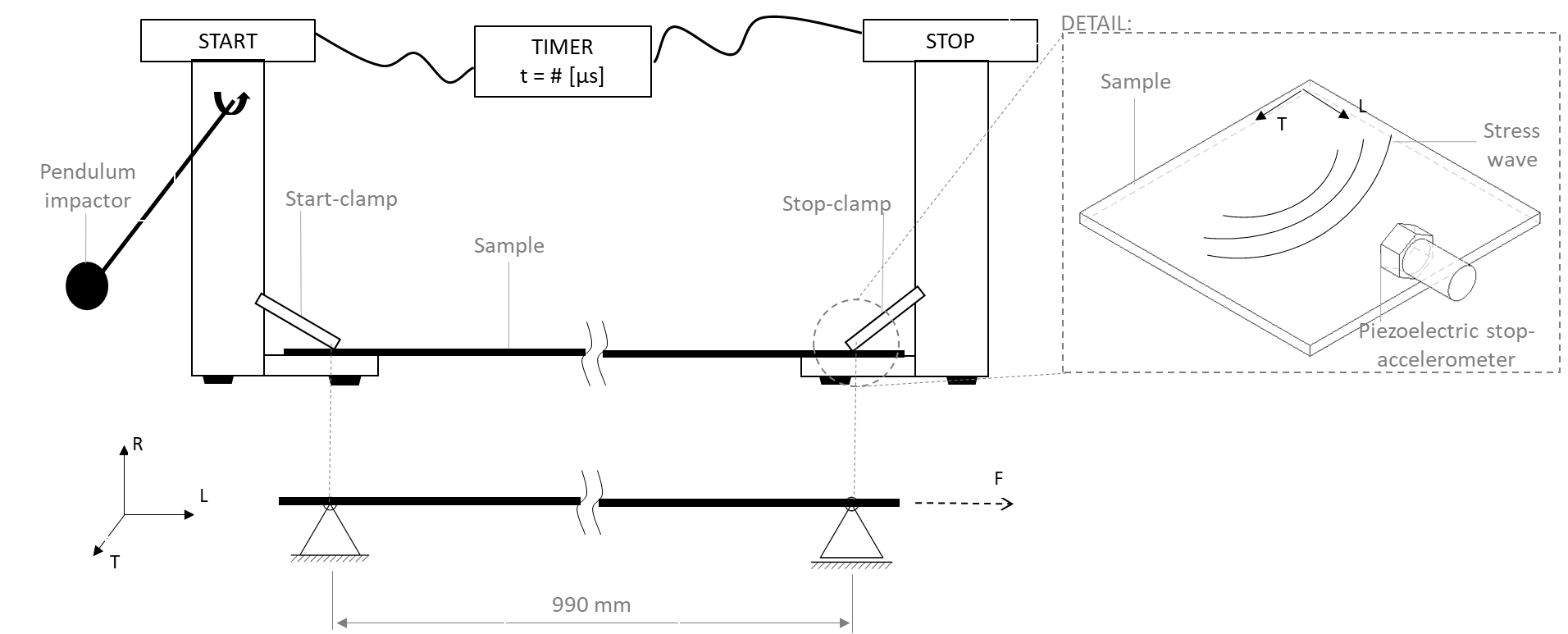Fig. 1. Schematic test setup for the METRIGUARD Model 239A portable stress wave timer.

(L= Longitudinal, R= Radial, T= Tangential, F= pre-force before testing)

(Own depiction based on (Metriguard A239A))

As depicted by the detail in Fig. 1, both piezoelectric accelerometers where mounted at the end of the sample perpendicular to the stress wave propagation direction. The start- and stop-clamps are adjustable in height and in clamp-pressure. The pendulum impactor is fixed in the frame profile on the start side. Both frame profiles are equipped with rubber supports on the bottom to minimize the vibrational influence from the working top that the clamps are set up on. The sample itself was elevated from the ground through the rubber supports and the frame profile itself. The dMOE was calculated according to Niemz and Sonderegger (2017) as shown in Eq. 2,

dMOE = ρv² (2)

where dMOE is the dynamic modulus of elasticity (GPa), ρ is the density (kg/m³), and v is the transmission speed (m/s). With respect to the test set up, two main things needed to be fulfilled in order to obtain reliable transmission times. The quartz piezoelectric crystal on both the start and the stop clamp needed to be in perfect contact with the sample. Both clamps for the Metriguard 239A had a tapered shape to guarantee good contact between the piezoelectric crystal and the sample. To prevent squeezing and failing of the wood cells at the clamping points of the thin veneers; the clamping pressure needed to be finely adjusted. Due to the small thickness of the veneers, however, a different clamping force, and thus a different contact at the clamping shoes need to be assumed.

The static modulus of elasticity of each sample was determined via longitudinal tensile experiments in accordance to standard DIN EN 789 (2005) by using a universal testing machine (Z100, Zwick/Roell, Ulm, Germany) with a cell capacity of 100 kN and a resolution of 0.06 N. Clamping the specimens between the sample holders resulted in a free span length of 700 mm. For 40 samples the elongation of the specimens was measured at three positions (the upper, middle, and lower part of the samples) during three tensile experiments. For 12 samples the elongation of the specimens was determined at ten intersecting positions. A distance of 100 mm was chosen for the mechanical extensometer (Makrosense, Zwick/Roell, Ulm, Germany). The specimens were loaded up to a peak force of 800 N and a stress-strain curve was recorded during the experiment. The sMOE was calculated afterwards according to standard DIN EN 789 (2005) via the regression method between 20% and 80% of the peak force, as shown in Eq. 3,

sMOE = (F2-F1)l1/(u2-u1)A (3)

where sMOE is the static modulus of elasticity (GPa), F– F1 is the force increase between 10% and 80% of Fmax (N), u– u1 is the deformation between 10% and 80% of Fmax obtained with linear regression (mm), l1 is the measuring length (mm), and A is the cross-sectional area of the sample (mm²). For comparison with the dMOE, the sMOE was taken as average of the three or ten areas respectively for each sample.

The tensile strength (σt) of the 40 samples with three elongation measurements was determined according to standard DIN 789 (2005). Prior to testing, the respective samples were reduced to a width of 40 mm ± 1 mm and a length of 250 mm ± 1 mm to fit the available clamps of the test setup. Mounting the samples in the setup resulted in a free testing length of 210 mm. The specimens were pre-loaded with 100 N to compensate for possible distortions due to conditioning. A testing speed of 2 mm/min was chosen to reach a testing time of 300 s ± 120 s. The test was stopped after a 30% force reduction was reached. The σt was calculated according to standard DIN EN 789 (2005), as shown in Eq. 4,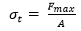(4)

where σt is the tensile strength (MPa), Fmax is the peak force (N), and A is the cross-sectional area of the sample (mm²). After testing, the average tensile strength was calculated from the three tested sample strips.

Microscopic Images

The fractured surfaces, as well as the fiber orientation of selected samples, were investigated via scanning electron microscopy (SEM) using a Hitachi TM3030 (Hitachi, Tokyo, Japan). Multiple areas along the yield line were cut out using a microdissection cutter and placed on a microscope slide. Microscopic pictures were taken using the corresponding software (Hitachi TM3030, Tokyo, Japan) and further processed using ImageJ (National Institute of Health, Bethesda, Maryland).

Post-sorting of Veneers

In order to investigate the influence of defects on the strength of veneers a post-sorting of the sample batch into three different grades was carried out after testing. The sorting was done visually labeling the veneers either grade A, B, or C corresponding to own grading rules: Grade A veneers did neither include knots nor high fiber deviations. Grade B veneers included at least one or more knots, and grade C veneers did show high fiber deviations. The fiber deviation was estimated visually, characterizing veneers with at least 15° fiber deviation in the longitudinal direction of the veneer as this could already decrease strength by 50% (Kollmann 1951). Figure 2 gives an example for each of the three grades.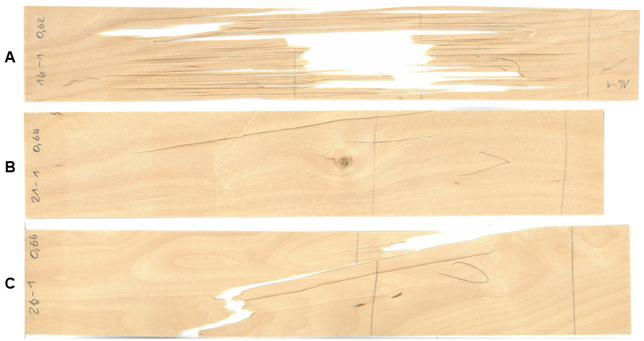Fig. 2. Example veneers for grade A (no defects), B (knots) and C (fiber deviation > 15°)

RESULTS AND DISCUSSION

Production of high-performance wood elements for new applications of wood in the area of mechanical and automotive engineering involved laminated structures (Jost et al. 2018; Müller et al. 2019). Knowing the dMOE of every single veneer gives the opportunity to estimate the dMOE, the sMOE, and even the modulus of rupture (MOR) of the whole element. This opens the possibility of developing new approaches for a safety concept. In addition, the grading and joining of the veneers with respect to their mechanical properties will improve the material efficiency of such elements.

Based on non-destructive testing, Koch and Woodson (1968) suggested pre-sorting the veneers for the production of LVL and tested pine veneers (0.5 mm x 60 mm x 2540 mm), which were used for the production of LVL beams (450 mm x 50 mm x 7620 mm). Veneers with a high dMOE were then used for the outer parts of the beam. The researchers reported a strong correlation (r = 0.919) between the dMOE and the sMOE for testing single veneers. However, a strong correlation (r = 0.888) was also observed during testing for the dMOE of single veneers and for measuring the dMOE and sMOE of the entire LVL beams. Jung (1982) also investigated the potential of the ultrasonic wave propagation method to pre-sort veneers (6 mm x 127 mm x 2640 mm) for the production of LVL. Comparable to Koch and Woodson (1968), Jung (1982) used the dMOE to predict the sMOE of the LVL beams. Correlation between the dMOE of the veneers and the sMOE of the LVL beams, which were tested via static bending, ranged from 0.659 to 0.879. Both studies (Koch and Woodson 1968; Jung 1982) established that the pre-sorting of veneers, based on the dMOE measurement, was a reliable method to predict the sMOE of LVL beams. Pu and Tang (1997) investigated the effects of relative humidity (RH) on the non-destructive testing method and measured the dMOE of veneers and LVL beams (38 mm x 89 mm x 2438 mm) at different RHs. Pu and Tang (1997) found that the dMOE decreased as the RH increased. Xue and Hu (2013) performed a study on two hardwood species, poplar (Populus ussuriensis Kom.) and birch wood (Betula platyphylla Suk.), and the dMOE and sMOE of different sized samples (25 mm x 25 mm x 575 mm and 25 mm x 90 mm x 575 mm) were investigated. Among other parameters, Xue and Hu (2013) measured the wave propagation time in the longitudinal direction of veneers and calculated the dMOE of LVL beams. Furthermore, Xue and Hu (2013) measured the sMOE of the LVL beams according to the Japanese agricultural standard of structural laminated veneer lumber (2017). In addition, the dMOE and sMOE were compared, and a strong correlation for poplar (r = 0.86 to 0.92) was found, but a weaker correlation was found for birch wood (r = 0.65 to 0.91). Further research on six hardwood species was done by McGavin (2015). Peeled veneers were acquired from plantation trees. An increase in the dMOE from the pith to bark was observed for the veneer samples with varying thickness (2.4 mm to 3 mm x 150 mm x 1300 mm). However, the literature research performed showed that measurements of the wave propagation time of thin hardwood veneers were lacking. The result presented hereafter attempt to close this gap.

Sample Properties

Table 1 summarizes the results for the density (ρ), aspect ratio (b/h), wave speed (v), tensile strength (σt), dynamic modulus of elasticity (dMOE), and static modulus of elasticity (sMOE).

Table 1. Overview of Investigated Properties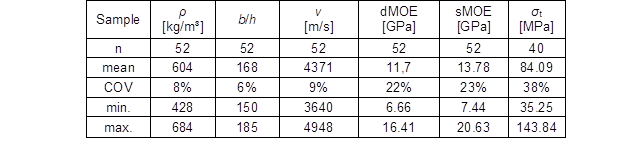The average density (ρ) was 604 kg/mwith a coefficient of variation (COV) of 8%, and the density values ranged from 428 kg/m3 to 684 kg/m³. The average value of the author’s measurements was slightly below the typical literature values for birch wood with a reference density of between 610 kg/mand 680 kg/m3 at 0% moisture content and between 650 kg/m3 and 730 kg/mat 15% moisture content (Sell 1989). A possible reason for this deviation could be that pre-sorted and defect-free samples usually are employed in typical literature as cited by Sell (1989). Leading to a higher mean density compared to an unsorted sample batch as used in this study. The mean aspect ratio (b/h) of the samples was 168 with a COV of 6%. Values ranged from 150 to 185. As described by Bucur (2006), the observed wave velocity decreased as the aspect ratio increased. The specimen length posed no influence on the observed velocity. The sound wave transmission experiments resulted in an average speed of 4371 m/s. The values ranged from 3640 m/s to 4948 m/s, which corresponded to a COV of 9%. According to investigations by Gerhards (1982), additional factors influencing wave velocity included fiber angle, fiber deviation in the vicinity of knots, wood temperature, moisture content, fungal decay, and the ratio of earlywood to latewood. The average dMOE was 11.70 GPa with a COV of 22%, and values ranged from 6.66 GPa to 16.41 GPa. The sMOE obtained through tensile tests yielded an average of 13.78 GPa with a COV of 23% and ranged from 7.44 GPa to 20.63 GPa. Therefore, the sMOE obtained via tensile testing was in the range of the typical literature values (13.3 GPa to 16.2 GPa) tested via three-point bending (Sell 1989). The tensile strength evaluated from 40 veneers divided into three sub-samples resulted in an average of 84.09 MPa with a COV of 38%. The values ranged from 35.25 MPa to 143.84 MPa. The average value of the author’s measurements was greatly below the typical literature values for birch wood tensile strength shown in Sell (1989) (130 MPa to 140 MPa). This was attributed primarily to the presence of defects within the veneers. Usually standard tests are carried out on defect free, fine grained samples with as little fiber deviation as possible. In comparison to other literature above, no pre-sorting of the material was carried out in these laboratory tests. Therefore, a certain level of defects could be expected in the sample batch, also influencing the resulting strength. In addition, the veneers were quite thin, and the size effect could have a major effect on the strength due to the local fiber deviation, especially under tensile load. Further discussion on the influence of these factors on the strength of veneers can be found in the section on “Dynamic Modulus of Elasticity and Strength”.

Table 2 shows an overview of the Pearson-correlation between the investigated properties. Strong correlation (r = 0.821) was achieved between the dMOE and the sMOE, as well as the dMOE and v (r = 0.942). Also notable was the positive correlation between the dMOE and the tensile strength (r = 0.665) and between the sMOE and v (r = 0.785).

Table 2. Pearson-correlations of Investigated Properties (n = 52)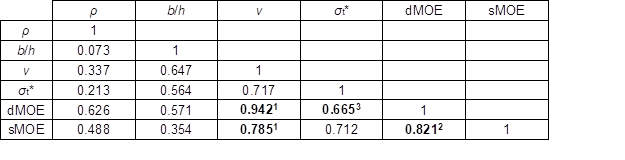* n = 40; = Fig. 2; = Fig. 6; = Fig. 7

Dynamic and Static Modulus of Elasticity

The relationship between the dMOE and the sound wave transmission time provided the most important information for estimating the sMOE.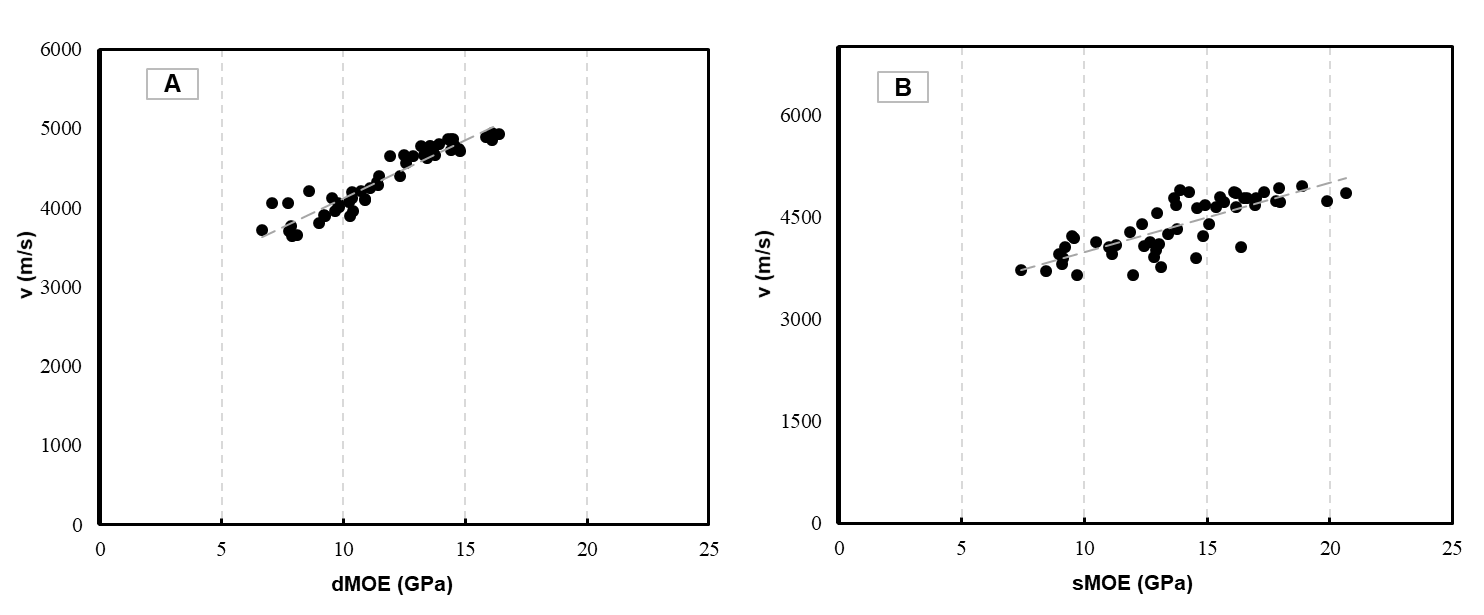Fig. 3. Correlation between the dMOE and v (A) and between the sMOE and v (B) of 52 samples

Analogous to the literature reviewed, it could also be stated for this thin veneer study that the correlation between the dMOE and sMOE and the sound wave transmission time was excellent and good, respectively (as shown in Fig. 3). For veneers with a thickness of approximately 0.5 mm, the correlations found during the study were confirmed.

Comparing pairwise, the measured sMOE typically showed a greater value than the dMOE in most cases (as shown in Fig. 4). For the entire sample set (n = 52), the sMOE was 2.08 GPa greater than the dMOE, which was an overestimate of approximately 14% for the sMOE. These results contradicted the results reported in the literature, which reported an underestimation of the sMOE. One reason could be that in the author’s case, the sMOE was measured in a tensile test, but in earlier studies the sMOE was determined via a 3-point bending test. The deflection of a bending beam resulted from the dimensions, as well as the shear modulus and modulus of elasticity according to standard EN 408 (2012). In the case of the LVL beams, the individual veneer layers were adhesively bonded, which led to a reduction in the shear modulus when compared to solid wood.

For LVL made out of birch wood, Xue et al. (2013) reported dMOE values which were greater than or equal to the sMOE values. Similar results were obtained by Hassan et.al. (2013) for pine, where the dMOE was approximately 1 GPa to 2GPa above the respective sMOE. In both cases, the proportion of deflection due to shear deformation was considered. This meant that for the calculation of the sMOE, the shear portion was subtracted. Nevertheless, the dMOE was higher than the sMOE, which meant that the shear deformation cannot be solely responsible for the differences in the values.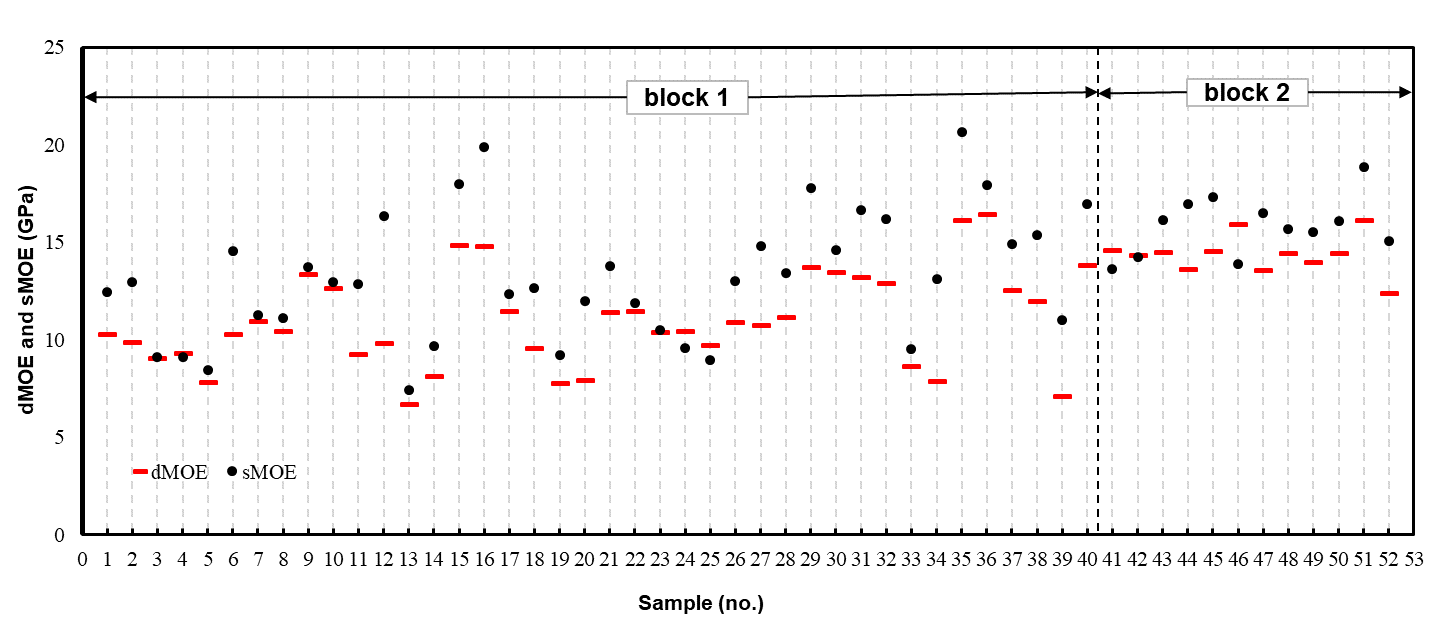Fig. 4. The dMOE (red bar) and sMOE (black dot) of the 52 samples. The sMOE was an average of three (1; samples 1 to 40) or ten (2; samples 41 to 52) measured areas within a veneer

A strain measurement for the sMOE over the entire specimen length was not possible due to experimental reasons (the maximum length of the extensometer was 100 mm). The sMOE was therefore measured at three points over the entire sample length. Due to local differences, the results showed a high variability in stiffness (as shown in Fig. 3, block 1). Using additional samples with sample numbers 41 to 52 (as shown in Fig. 4, block 2 and Fig. 5), it was shown that with multiple measurements over the sample length, the scattering of the sMOE values was drastically decreased, since the individual extreme values were no longer as pronounced. For samples 1 to 40 (block 1) the sMOE was 2.25 GPa greater than the dMOE (16% higher), whereas for samples 41 to 52 (block 2), the sMOE was only 1.5 GPa greater than the dMOE (approximately 9% higher). For statistical reasons, a further increase in the number of sMOE measurements would hardly change this result. This meant, by using the existing equipment for the determination of the dMOE would in any case would result in an underestimation in comparison to the sMOE, which conflicted with former results.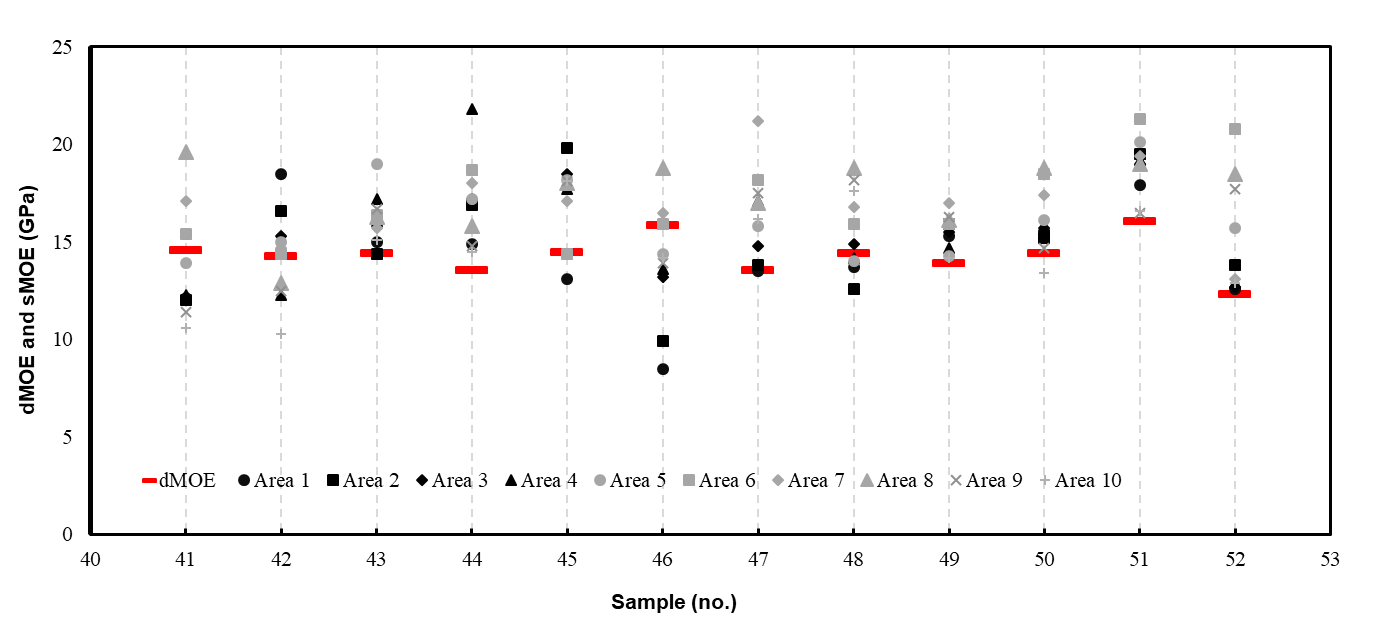Fig. 5. Comparison between the dMOE (red bar) of the complete veneer and the sMOE of the single measuring areas (1 to 10) of 12 samples

All factors that led to a damping of the sound waves also influenced the sound wave transmission time (Bucur 2006). Therefore, it was assumed that the low material thickness (0.5 mm), wood defects, such as knots, cracks, fiber deviations, etc., and the experimental setup itself were further reasons for the differences. According to Bucur (2006) the wave transmission time decreased with an increase in the aspect ratio of width (b) to thickness (h) of the sample, whereas the length was not biasing the result. In the study by Bucur (2006) the aspect ratio (b/h) was varied and reached a maximum value of 14. The results showed a trend towards a lower dMOE value as the aspect ratio increased. However, the maximum aspect ratio for the author’s measurements was 168, which meant that the low veneer thickness at least partly explained the drastically lower dMOE values compared to the other studies. Koch et al. (1968) found similar aspect ratios in samples similar to the author’s measurements, but no information concerning the differences in the dMOE and sMOE were provided in the publication. Figure 6 shows the different positions of the defects and fiber deviations of the veneers. It can be assumed that the veneers showed higher elongation in the vicinity of these regions, which would influence the sMOE measurements. It can be furthermore assumed that the first cracks would initiate in the regions with the highest fiber deviation. For the dMOE measurements, it could be assumed that the position of the defects would have almost no effect. In sample A (as shown in Fig. 6), the area where the fracture was initiated was near a knot. This area was clearly inside the area where the sMOE was measured via an extensometer. Therefore, this sample had a relatively low (9.13 GPa) sMOE and showed a better agreement with the 9.25 GPa dMOE measurement. In contrast, the fracture zone of sample B (as shown in Fig. 6) was completely outside the measuring range for the determination of sMOE. Therefore, the sMOE of this sample (14.53 GPa) was drastically higher than the dMOE value (10.26 GPa).